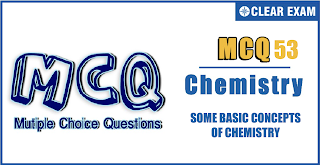## [LATEST]\$type=sticky\$show=home\$rm=0\$va=0\$count=4\$va=0

IIT JEE exam which consists of JEE Main and JEE Advanced is one of the most important entrance exams for engineering aspirants. The exam is held for candidates who are aspiring to pursue a career in the field of engineering and technical studies. Chemistry is important because everything you do is chemistry! Even your body is made of chemicals. Chemical reactions occur when you breathe, eat, or just sit there reading. All matter is made of chemicals, so the importance of chemistry is that it's the study of everything..

Q1.40 mL of 0.05 M solution of sodium sesquicarbonate(Na_2 CO_3 NaHCO_3;2H_2 O) is titrated against 0.05 M HCl. x mL of HCl is used when phenolphthalein is the indicator and y mL of HCl is used when methyl orange is the indicator in two separate titrations. Hence e(y-x) is
•  80 mL
•  30 mL
•  120 mL
•  none
Solution
(a) 0.05 M Na_2 CO_3=1 N Na_2 CO_3 0.05 M NaHCO_3=0.05 N NaHCO_3 40 L of 0.1 Na_2 CO_3=40 mL of 0.1 N HCl (For complete reaction)=80 mL of 0.05 M HCl For 50% reaction =40 mL of 0.05 M HCl With phenolphthalein =x=40 mL 40 mL of 0.05 NaHCO_3=40 mL of 0.05 M HCl With methyl orange, y=80+40=120 mL ∴(y-x)=80 mL

Q2.A suspension containing insoluble substances ZnS,CuS,HgS, and FeS, is treated with 2N HCl. On filtering, the filtrate contained appreciable amounts of which one of the following groups?
•  Zinc And Mercury
•  Silver And Iron
•  Copper And Mercury
•  Zinc,Copper And Iron
Solution

(d) On adding 2NHCl,AgCl and HgCl_2 are precipitated. Filtrate will contain Zn^(2+),Cu^(2+) and Fe^(2+)

Q3.   A sodium hydroxide solution has pH=12.(pH=log⁡〖1/((H+ ) )〗 ) 100 mL of this solution has NaOH
•  0.4 g
•  0.04 g
•  0.004 g
•  4 g
Solution
(b) pH=12 ∴[H+ ]=10(-12) M [OH- ]=10(-2) M=10(-2) mol L(-1) =10(-3) mol per 100 mL =10(-3)×40 g NaOH per 100 mL =0.04 g

Q4. The volume strength of 1.5 N H_2 O_2 solution is
•  4.8
•  8.4
•  3.0
•  8.0
Solution
(b) 2H_2 O_2→2H_2 O_ +O_2 68 g 22.4 L (at STP) Mass of H_2 O_2 in 1.5 N solution =Ew of H_2 O_2×1.5 N =17×1.5=25.5 g So, volume strength of 1.5 N H_2 O_2 solution =(22.4 L ×25.5 g)/68.0=8.4 L

Q5.What volume of 0.1 M KMnO_4 is needed to oxidise 5 mg of ferrous oxalate in acidic medium (Mw of ferrous oxalate is 144)
•  0.20 mL
•  0.1 mL
•  0.4 mL
•  2.08 mL
Solution
(a) mEq of KMnO_4=V×0.1×5 mEq of Fe(C_2 O_4 )_2=(Weight in g)/Ew×1000 [█(Fe^(+2) →Fe^(+3)+e^-@C_2 O_4^( 2-)→2CO_2+2e^- )](n=3) Ew of Fe(C_2 O_4 )=Mw/3=144/3 ∴mEq of Fe(C_2 O_4 )=(5×10^(-3))/(144/3)×10^3=(5×3)/144 ∴mEq of KMnO_4=mEq of Fe(C_2 O_4 ) ∴V×0.1×5=(5×3)/144 ∴V=0.20 mL

Q6. 5 mL of N-HCl, 20 mL of N/2 H_2 SO_4 and 30 mL of N/3 HNO_3 are mixed together and the volume is made to 1 L. The normality of the resulting solution is
•  n/5
•  n/10
•  n/20
•  n/40
Solution
(d) N_1 V_1+ N_2 V_2+ N_3 V_3=N_4 V_4 (V_4=V_1+ V_2+ V_3+ V_4 ) or V_4 = Final volume = 1L ≡ 1000 mL 5×N+20×N/2+ 30×N/3=N_4×1000 ∴N_4=N/40

Q7.What volume of H_2 at 273 K and 1 atm will be consumed in obtaining 21.6 g of elemental boron (atomic mass of B = 10.8) from the reduction of BCl_3 with H_2
•  89.6 L
•  67.2 L
•  44.8 L
•  22.4 L
Solution
(b) 2 BCl_3+ 3H_2→2B+6HCl 2×10.8 g B≡3×22.4 L of H_2 21.6 g B ≡(3×22.4×21.6)/(2×10.8)=67.2 L of H_2

Q8.By dissolving 1 mole each of the following acids in 1 L water, the acid which does not give a solution of 1 N strength is
•  H3PO4
•  HCLO4
•  HNO3
•  HCL
Solution
(a) H3PO4 Is a tribasic acid

Q9.To prepare a solution of concentration of 0.03 g/mL of AgNO_3, what amount of AgNO_3should be added in 60 mL of solution?
•  1.8 g
•  0.8 g
•  0.18 g
•  None of these
Solution
(a) Concentration in terms of g/mL is given as 0.03=(mass of AgNO_3)/60 Mass of AgNO_3=60×0.03=1.8 g

Q10.10 mL of 0.2 N HCl and 30 mL of 0.1 N HCl together exactly neutralizes 40 mL of solution of NaOH, which is also exactly neutralised by a solution in water of 0.61 g of an organic acid. What is the equivalent weight of the organic acid?
•  61
•  91.5
•  122
•  183
Solution
(c) 10 mL of 0.2 N HCl +30 mL of 0.1 N HCl ≡40 mL of NaOH (≡0.61 g organic acid) mEq of HCl≡mEq of NaOH≡mEq of organic acid 10×0.2 +30×0.1 ≡0.61/E×1000 5=(0.61×1000)/E E=610/5=122#### Written by: AUTHORNAME

AUTHORDESCRIPTION## Want to know more

Please fill in the details below:

## Latest NEET Articles\$type=three\$c=3\$author=hide\$comment=hide\$rm=hide\$date=hide\$snippet=hide

Name

ltr
item
BEST NEET COACHING CENTER | BEST IIT JEE COACHING INSTITUTE | BEST NEET & IIT JEE COACHING: SOME BASIC CONCEPTS OF CHEMISTRY Quiz-53
SOME BASIC CONCEPTS OF CHEMISTRY Quiz-53
https://1.bp.blogspot.com/-CGkXLjXHoZE/YOkmWbnvC1I/AAAAAAAAVlM/5PgWqIHomqUvJ7NKvpnFeJfUWZH_Bpl6ACLcBGAsYHQ/s320/Screenshot%2B2021-07-06%2Bat%2B6.35.18%2BPM.png
https://1.bp.blogspot.com/-CGkXLjXHoZE/YOkmWbnvC1I/AAAAAAAAVlM/5PgWqIHomqUvJ7NKvpnFeJfUWZH_Bpl6ACLcBGAsYHQ/s72-c/Screenshot%2B2021-07-06%2Bat%2B6.35.18%2BPM.png
BEST NEET COACHING CENTER | BEST IIT JEE COACHING INSTITUTE | BEST NEET & IIT JEE COACHING
https://www.cleariitmedical.com/2021/07/some-basic-concepts-of-chemistry-quiz-53.html
https://www.cleariitmedical.com/
https://www.cleariitmedical.com/
https://www.cleariitmedical.com/2021/07/some-basic-concepts-of-chemistry-quiz-53.html
true
7783647550433378923
UTF-8

STAY CONNECTED